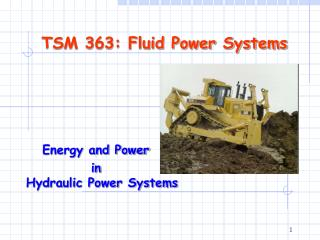DownloadDownload PresentationTSM 363: Fluid Power Systems

# TSM 363: Fluid Power Systems

Télécharger la présentation## TSM 363: Fluid Power Systems

- - - - - - - - - - - - - - - - - - - - - - - - - - - E N D - - - - - - - - - - - - - - - - - - - - - - - - - - -
##### Presentation Transcript

1. TSM 363: Fluid Power Systems Energy and Power in Hydraulic Power Systems

2. Goals of this Lecture • Fundamentals of Hydraulic Power Transmission • Multiplication of force • Conservation of energy • Continuity of fluids • Energy and Power in Hydraulic Systems • Energy conversion in hydraulic systems • Hydraulic power and efficiency

3. Fluid Power Energy Transmission • vo, Fo • P1,Q1 • P4 Q4 • ni, Ti • M PM-in PH-in • no, To PH-out PM-out • EnergyLevel

4. Critical Facts of Hydraulic Systems • Power is transmitted by pushing pressurized liquids in a confined system. • Liquids form in the shape of the container with equal pressure in all directions. • Hydraulic fluids can be considered incompressible.

5. 100 N 10 N 1 m2 10 m2 10 N/m2 Multiplication of Force Pascal’s Law

6. 8836 lbf Try This Together! • A hydraulic cylinder has a diameter of 2.5 inches. Compute the area of the piston. • What pressure is developed lifting a 8836 lbf load? • What is the pressure in Pascals?

7. Solutions

8. Conservation of Energy Bernoulli’s equation

9. Conservation of Energy (Cont.) Orifice equation

10. Example The pressure drop between the upstream flow and the in-orifice flow as shown above is 1.0 MPa. Compare the flow rate passing through the orifice when a sharp-edged orifice of 10 mm in diameter (Assume the specific fluid gravity is 900 kg/m3).

11. Solutions

12. Continuity of Fluids flow continuity equation

13. Try It by Yourself After Class For a pipeline of D1=25mm, D2=15mm, and v1=2.0m/s, try to find (a) the volumetric flow rate Q, and (b) the fluid velocity at cross section 2. Hint: According to the fluid continuity principle, the volumetric flow rate should be the same regardless of the size of the pipe cross section if the fluid between the two cross sections is confined.

14. Energy Conversion in Hydraulic Systems

15. Mechanical and Hydraulic Power Mechanical power Hydraulic power

16. Example and Try It Together A hydraulic pump delivers pressurized fluid at 50 L/min and 9,000 kPa. How much hydraulic power can this pump deliver? If the overall efficiency of this pump is 90%, how much mechanical power is needed to drive the pump? If all the pressurized fluid is delivered to a motor to drive a load, what is the maximum useful power the motor can deliver if the motor has the same overall efficiency as the pump?

17. (a) The hydraulic power the pump can deliver: (b) The mechanical power needed to drive the pump: (c) The mechanical power available at the motor for driving the load:

18. Lecture Summary • Discussed a few basic theoretical equations, and their applications in hydraulic systems: • Multiplication of force • Conservation of energy • Continuity of fluids • Hydraulic power and efficiency